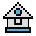Home

# RND Function

#### Purpose:

To return a random number between 0 and 1.

#### Syntax:

`RND[(x)]`

The same sequence of random numbers is generated each time the program is run unless the random number generator is reseeded (see RANDOMIZE statement). If x is equal to zero, then the last number is repeated.

If x is greater than 0, or if x is omitted, the next random number in the sequence is generated.

To get a random number within the range of zero through n, use the following formula:

`INT(RND*(n+1))`

The random number generator may be seeded by using a negative value for x.

#### Examples:

```10 FOR I=1 TO 5
20 PRINT INT(RND*101);
30 NEXT
RUN
Ā53 30 31 51 5```

Generates five pseudo-random numbers within the range of 0-100.